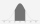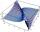Kerosine and petrol

if 4 litres of petrol containing 15% kerosine are added to another 7 litres of petrol containing 10% kerosine, what percentage of the petrol is kerosine?

Result

p =  11.818 %

Solution:Leave us a comment of this math problem and its solution (i.e. if it is still somewhat unclear...):Be the first to comment!To solve this verbal math problem are needed these knowledge from mathematics:

Looking for help with calculating arithmetic mean? Looking for a statistical calculator? Our percentage calculator will help you quickly calculate various typical tasks with percentages.

Next similar math problems:

1. Final examThere are 5 learners in a class that has written a final exam Aleta scored 55% vera scored 36% and Sibusiso scored 88% if Thoko scored 71% and the class average was 63%. What was Davids score as a percentage?
2. Normal DistributionAt one college, GPA's are normally distributed with a mean of 3.1 and a standard deviation of 0.4. What percentage of students at the college have a GPA between 2.7 and 3.5?
3. A perineumA perineum string is 10% shorter than its original string. The first string is 24, what is the 9th string or term?
4. Theorem proveWe want to prove the sentence: If the natural number n is divisible by six, then n is divisible by three. From what assumption we started?
5. CarpentersCarpenters 1 and 2 spend 10 days and 5 days respectively to make one table. If 50 tables were made by the first carpenter and 30 tables were made by the second carpenter, What is the average time spent on the products?
6. AverageIf the average(arithmetic mean) of three numbers x,y,z is 50. What is the average of there numbers (3x +10), (3y +10), (3z+10) ?
7. Life expectancyThe life expectancy of batteries has a normal distribution with a mean of 350 minutes and standard deviation of 10 minutes. What the range in minutes 68% of the batteries will last? What is the range in minutes approximately 99.7% of batteries will last?
8. Here isHere is a data set (n=117) that has been sorted. 10.4 12.2 14.3 15.3 17.1 17.8 18 18.6 19.1 19.9 19.9 20.3 20.6 20.7 20.7 21.2 21.3 22 22.1 22.3 22.8 23 23 23.1 23.5 24.1 24.1 24.4 24.5 24.8 24.9 25.4 25.4 25.5 25.7 25.9 26 26.1 26.2 26.7 26.8 27.5 27.6
9. Simple interest 3Find the simple interest if 11928 USD at 2% for 10 weeks.
10. 75th percentile (quartille Q3)Find 75th percentile for 30,42,42,46,46,46,50,50,54
11. Median and modusRadka made 50 throws with a dice. The table saw fit individual dice's wall frequency: Wall Number: 1 2 3 4 5 6 frequency: 8 7 5 11 6 13 Calculate the modus and median of the wall numbers that Radka fell.
12. Standard deviationFind standard deviation for dataset (grouped data): Age (years) No. Of Persons 0-10 15 10-20 15 20-30 23 30-40 22 40-50 25 50-60 10 60-70 5 70-80 10
13. Harmonic and arithmetic meansThe local Utah Department of Child Service office wants to project staffing needs based on current social worker assignments. They have the number of cases per social worker for the following staff: Mary: 25 John: 35 Ted: 15 Lisa: 45 Anna: 20 Calculat
14. Geometric progression 2There is geometric sequence with a1=5.7 and quotient q=-2.5. Calculate a17.
15. Box and whisker plotConstruct a box and whisker plot for the given data. 56, 32, 54, 32, 23, 67, 23, 45, 12, 32, 34, 24, 36, 47, 19, 43
16. AS sequenceIn an arithmetic sequence is given the difference d = -3 and a71 = 455. a) Determine the value of a62 b) Determine the sum of 71 members.
17. AverageThe arithmetic mean of the two numbers is 71.7. One number is 5. Calculate the second number.